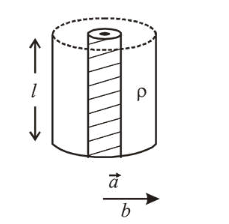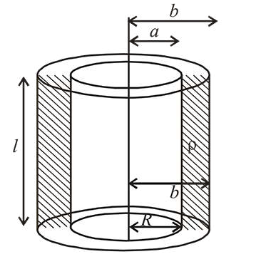# Model a torch battery of length l to be made up of a thin cylindrical barQuestion:

Model a torch battery of length $l$ to be made up of a thin cylindrical bar of radius ' $a$ ' and a concentric thin cylindrical shell of radius ' $b$ ' filled in between with an electrolyte of resistivity $\rho$ (see figure). If the battery is connected to a resistance of value $R$, the maximum Joule heating in $R$ will take place for:1. (1) $R=\frac{\rho}{2 \pi l}\left(\frac{b}{a}\right)$

2. (2) $R=\frac{\rho}{2 \pi l} \ln \left(\frac{b}{a}\right)$

3. (3) $R=\frac{\rho}{\pi l} \ln \left(\frac{b}{a}\right)$

4. (4) $R=\frac{2 \rho}{\pi l} \ln \left(\frac{b}{a}\right)$

Correct Option: , 2

Solution:

(2) Maximum power in external resistance is generated when it is equal to internal resistance of battery i.e., $P_{R}$ maximum when $r=R$

The maximum Joule heating in $R$ will take place for, the resistance of small element

$\Delta R=\frac{\rho d r}{2 \pi r l} \Rightarrow R=\frac{\rho}{2 \pi l} \int_{a}^{b} \frac{d r}{r}$or, $R=\frac{\rho}{2 \pi l} \ln \frac{b}{a}$